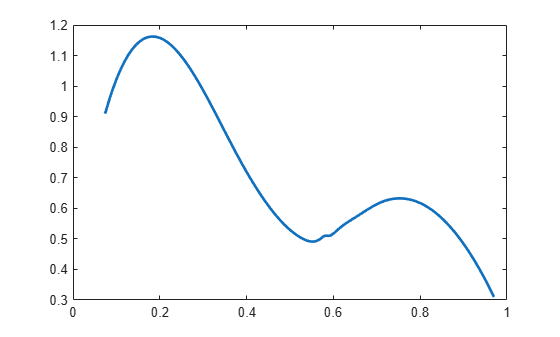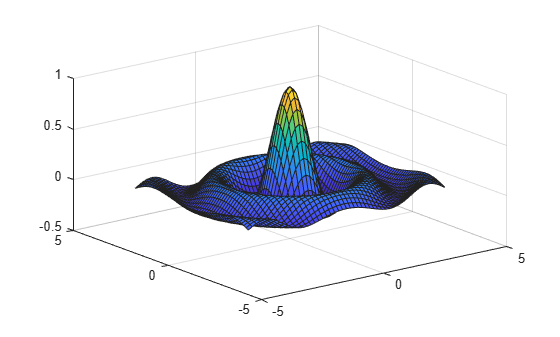fnval

Evaluate spline function

Description

example

v = fnval(f,x) provides the value f(x) at the points in x of the spline function f whose description is contained in f.

If f is scalar-valued and univariate, the output v is obtained by replacing each entry of x by the value of f at that entry. This is the intent in all other cases, except that, for a d-valued m-variate function, d-vectors replaces m-vectors.

For a univariate f :

• If f is scalar-valued, then v is of the same size as x.

• If f is [d1,...,dr]-valued, and x has size [n1,...,ns], then v has size [d1,...,dr, n1,...,ns], with v(:,...,:, j1,...,js) the value of f at x(j1,...,js), – except that:

• n1 is ignored if it is 1 and s is 2, i.e., if x is a row vector;

• MATLAB® ignores any trailing singleton dimensions of x.

For an m-variate f with m>1, with f [d1,...,dr]-valued, x might be either an array, or else a cell array {x1,...,xm}.

• If x is an array, of size [n1,...,ns], then n1 must equal m, and v has size [d1,...,dr, n2,...,ns], with v(:,...,:, j2,...,js) the value of f at x(:,j2,...,js), – except that:

• d1, ..., dr is ignored in case f is scalar-valued, i.e., both r and n1 are 1;

• MATLAB ignores any trailing singleton dimensions of x.

• If x is a cell array, then it must be of the form {x1,...,xm}, with xj a vector, of length nj, and, in that case, v has size [d1,...,dr, n1,...,nm], with v(:,...,:, j1,...,jm) the value of f at (x1(j1), ..., xm(jm)), – except that d1, ..., dr is ignored in case f is scalar-valued, i.e., both r and n1 are 1.

If f has a jump discontinuity at x, then the value f(x +), i.e., the limit from the right, is returned, except when x equals the right end of the basic interval of the form; for such x, the value f(x–), i.e., the limit from the left, is returned.

fnval(x,f) is the same as fnval(f,x).

fnval(...,'l') treats f as continuous from the left. This means that if f has a jump discontinuity at x, then the value f(x–), i.e., the limit from the left, is returned, except when x equals the left end of the basic interval; for such x, the value f(x +) is returned.

If the function is multivariate, then the above statements concerning continuity from the left and right apply coordinate wise.

Examples

collapse all

This example shows how to interpolate some data and plot and evaluate the resulting functions.

Define some data.

x = [0.074 0.31 0.38 0.53 0.57 0.58 0.59 0.61 0.61 0.65 0.71 0.81 0.97];
y = [0.91 0.96 0.77 0.5 0.5 0.51 0.51 0.53 0.53 0.57 0.62 0.61 0.31];

Interpolate the data and plot the resulting function, f.

f = csapi( x, y )
f = struct with fields:
form: 'pp'
breaks: [0.0740 0.3100 0.3800 0.5300 0.5700 0.5800 0.5900 0.6100 ... ]
coefs: [11x4 double]
pieces: 11
order: 4
dim: 1

fnplt( f )Find the value of the function f at x = 0.5.

fnval( f, 0.5 )
ans = 0.5294

Find the value of the function f at 0, 0.1, ..., 1.

fnval( f, 0:0.1:1 )
ans = 1×11

0.3652    1.0220    1.1579    0.9859    0.7192    0.5294    0.5171    0.6134    0.6172    0.4837    0.2156

Create a function f2 that represents a surface.

x = 0.0001+(-4:0.2:4);
y = -3:0.2:3;
[yy, xx] = meshgrid( y, x );
r = pi*sqrt( xx.^2+yy.^2 );
z = sin( r )./r;
f2 = csapi( {x,y}, z );

Plot the function f2.

fnplt( f2 )
axis( [-5, 5, -5, 5, -0.5, 1] );Find the value of the function f2 at x = -2 and y = 3.

fnval( f2, [-2; 3] )
ans = -0.0835

Input Arguments

collapse all

Spline function that you want to evaluate, specified as an object.

Points at which you want to evaluate the spline function f, specified as a vector, matrix or cell array.

Output Arguments

collapse all

Value f(x) at the points in x of the spline function f, returned as a scalar, vector, matrix or cell array.

Algorithms

For each entry of x, the function determines the relevant break-interval or knot-interval and assembles the relevant information. Depending on whether f is in ppform or in B-form, nested multiplication or the B-spline recurrence (see, e.g., [PGS; X.(3)]) are then used vector-fashion for the simultaneous evaluation at all entries of x. Evaluation of a multivariate polynomial spline function takes full advantage of the tensor product structure.

Evaluation of a rational spline follows up evaluation of the corresponding vector-valued spline by division of all but its last component by its last component.

Evaluation of a function in stform makes essential use of stcol, and tries to keep the matrices involved to reasonable size.